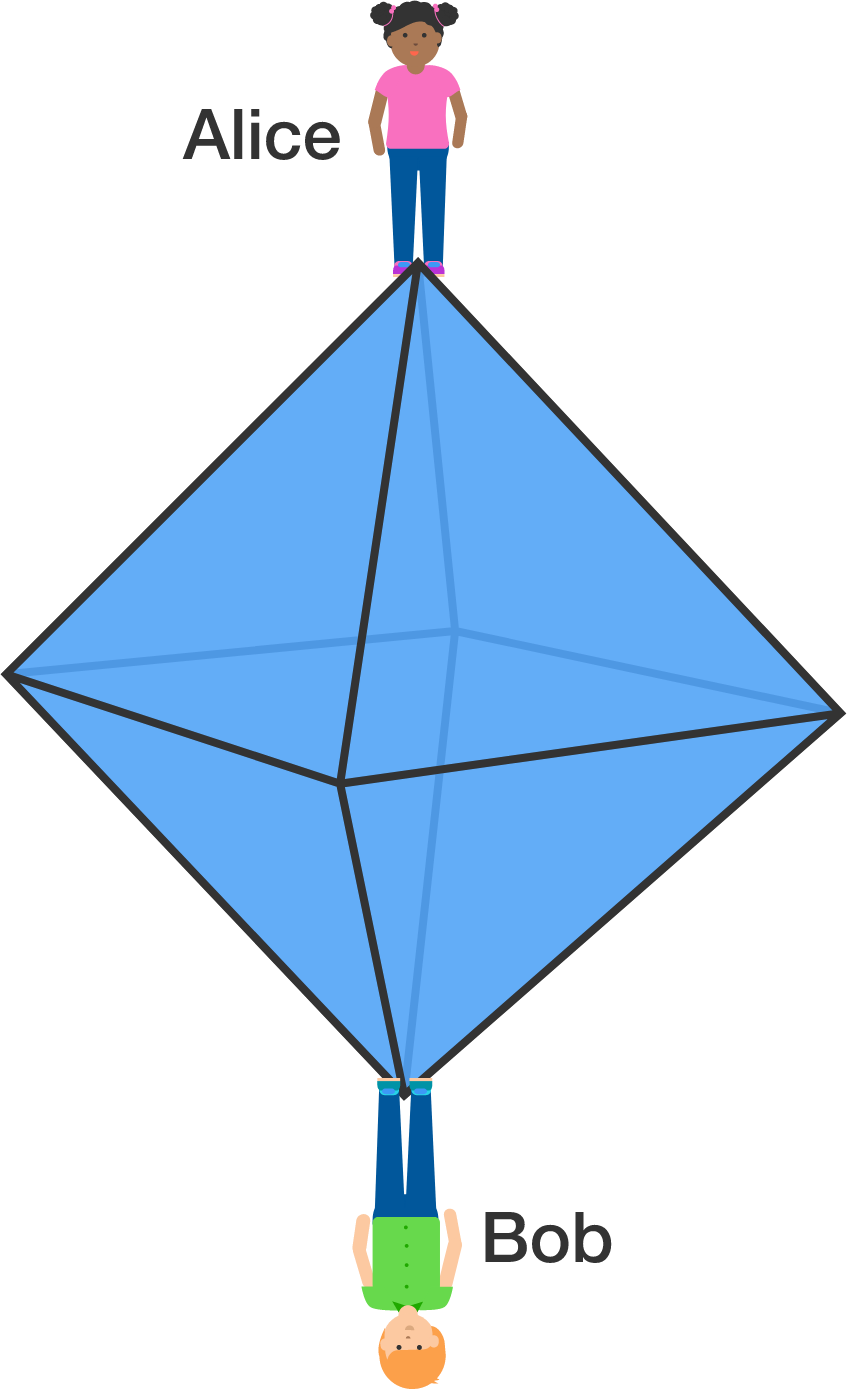# Lost on an Octahedron

Probability Level 3Alice and Bob stand on opposite vertices of a regular octahedron. At the beginning of every minute,

• each chooses an adjacent vertex uniformly at random and moves towards it;
• each moves at a constant rate of 1 edge per minute;
• they will stop when they meet up.

The expected value of the number of minutes until they meet up is equal to $\frac{p}{q}$, where $p$ and $q$ are coprime positive integers.

Find the value of $p + q.$


Note: It is possible that they would meet at a vertex or at the midpoint of an edge.

×

Problem Loading...

Note Loading...

Set Loading...